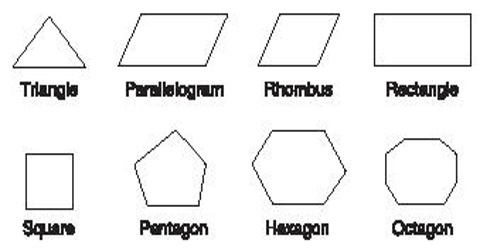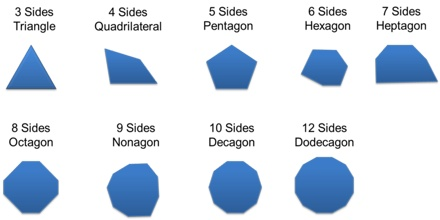Mathematic

# Polygons: Definition and TypesPolygons: Definition and Types

Polygons are plane figures with at least three straight sides and angles, and typically five or more. Triangles, quadrilaterals, pentagons, and hexagons are all examples of polygons. For example, a triangle has three sides, and a quadrilateral has four sides. So, any shape that can be drawn by connecting three straight lines is called a triangle, and any shape that can be drawn by connecting four straight lines is called a quadrilateral.Some brief examples of polygons:

Triangle: It is a plane figure with three straight sides and three angles. All triangles are convex and bicentric. That portion of the plane enclosed by the triangle is called the triangle interior, while the remainder is the exterior.

Quadrilaterals: It is a flat shape with four straight sides and four vertices. You can make a quadrilateral by taking anything straight and thin you might have handy: pens, toothpicks, chopsticks, etc. A square is one type of a special quadrilateral.

Pentagon: It is a plane figure with five straight sides and five angles. Pentagons can be regular or irregular and convex or concave.

Hexagon: It is a plane figure with six straight sides and angles. A hexagon is a 6-sided, 2-dimensional geometric figure. All of the sides of a hexagon are straight, not curved.

Heptagon: It is a plane figure with seven straight sides and angles. It also has seven vertices, or corners where sides meet, and seven angles. The angles are always found at the vertices.

Octagon: It is a plane figure with eight straight sides and eight angles. Octagons must have straight sides that connect; they cannot be curved or disconnected.

Nonagon: it is a plane figure with nine straight sides and nine angles. A regular nonagon is a nine-sided shape with equal sides and equal angles of 140 degrees each.

Decagon: It is a plane figure with ten straight sides and angles. It also has ten vertices – which is where the sides meet – and ten angles. The regular decagon is probably the most recognizable one. It’s called a regular decagon because all its sides and angles are congruent, or equal in size.